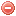# Description

The Mark 5 forest fire danger index ($$FFDI$$) was developed by McArthur (1967) in order to assess fire danger and behavior in eucalypt forest fuel types and has been widely used in Eastern Australia (Noble et al. 1980, Sharples et al. 2009a). The $$FFDI$$ requires temperature, relative humidity, wind speed and a fuel availability index (i.e. a drought factor) measured at 15:00 as input variables (Matthews 2009).

The drought factor is obtained based on soil moisture deficit (calculated using the
$$KBDI$$), time since last rain and rainfall amount. It ranges from 1 to 10, with a value of 10 indicating that a maximal amount of fuel is available for combustion (Matthews 2009, Sharples et al. 2009a).

# Formula

The $$FFDI$$ is defined as follows:

$FFDI=2\cdot e^{-0.45+0.987\cdot{ln (DF)}-0.0345\cdot{H_{15}}+0.0338\cdot{T_{15}}+0.0234\cdot{U_{15}}}$

where$$H_{15}$$ s relative air humidity [%], $$T_{15}$$ air temperature [°C], $$U_{15}$$ windspeed [km/h] and $$DF$$ a drought factor expressed as a function of the
$$KBDI_{SI}$$ (NB: in millimeters) and defined as follows (Noble et al. 1980):

$DF= min \Bigg[10,\dfrac{0.191\cdot{(KBDI_{SI}+104)}\cdot{(w+1)^{1.5}}}{3.52\cdot(w+1)^{1.5}+P-1}\Bigg]$

where $$w$$ is the number of days since last rain and $$P$$ the last precipitation amount [mm] (measured over the entire period of rainfall).

Griffith (1999) improved the calculation of the drought factor ($$DF$$) with following formulation (in Finkele et al. 2006):

$DF= min \Bigg[10, 10.5 \cdot \bigg( 1 - e^{-\frac{KDBI_{SI}+30}{40}}\bigg) \cdot \dfrac{41x^2 + x}{40x^2+x+1}\Bigg]$

where $$x$$ is

$x= \begin{cases} \dfrac{w^{1.3}}{w^{1.3}+P-2} & \text{if } w \geqslant 1 \text{ and } P > 2 \text{ mm}\\ \dfrac{0.8^{1.3}}{0.8^{1.3}+P-2} & \text{if } w =0 \text{ and } P > 2 \text{ mm}\\ 1 & \text{if } P < 2 \text{ mm}\\ \end{cases}$

where $$w$$ is the event age (defined as the number of days since the day with the largest daily rainfall amount within the rain event, Finkele 2006 referring to Sullivan 2001) and $$P$$ is the rainfall event amount in mm (sum of rainfall within the event).
The rainfall event is defined as consecutive days with rainfall amount above 2 mm.
The calculation of $$w$$ and $$P$$ only the past 20 days' rainfall is considered.

Based on the experiences in operational use, the Bureau of Meteorology further improved this Griffith formulation, proposing following limitation of $$x$$ (Finkele et al. 2006):

$x_{lim}= \begin{cases} \dfrac{1}{1+0.1135 \cdot KBDI_{SI}} & \text{if } KBDI_{SI} < 20 \\ \dfrac{75}{270.525 - 1.267 \cdot KBDI_{SI}} & \text{if } KBDI_{SI} \geqslant 20\\ \end{cases}$

The $$FFDI$$ is supposed to be calculated on a daily basis. The meteorological data used for its calculation have to be recorded at 3 pm (Matthews 2009).

No particular values or conditions are stipulated for starting the $$FFDI$$ calculation.

# Index interpretation

Fire agencies to simplify the interpretation of the $$FFDI$$ generally give following interpretation (Lucas 2005):

FFDI range Fire danger class
0 - 5 low
5-12 moderate
12-25 high
25-50 very high
> 50 extreme

Original publication:
McArthur (1967)

Other publications:
Noble et al. (1980)
Griffith (1999)
Lucas (2005)
Matthews (2009)
Sharples et al. (2009a)

### Bibliography SearchNo results!

No results were found. Are you sure you searched for a tag?

### Symbols

 Variable Description Unit $$T$$ air temperature °C $$T_{dew}$$ dew point temperature °C $$H$$ air humidity % $$P$$ rainfall mm $$U$$ windspeed m/s $$w$$ days since last rain (or rain above threshold) d $$rr$$ days with consecutive rain d $$\Delta t$$ time increment d $$\Delta{e}$$ vapor pressure deficit kPa $$e_s$$ saturation vapor pressure kPa $$e_a$$ actual vapor pressure kPa $$p_{atm}$$ atmospheric pressure kPa $$PET$$ potential evapotranspiration mm/d $$r$$ soil water reserve mm $$r_s$$ surface water reserve mm $$EMC$$ equilibrium moisture content % $$DF$$ drought factor - $$N$$ daylight hours hr $$D$$ weighted 24-hr average moisture condition hr $$\omega$$ sunset hour angle rad $$\delta$$ solar declination rad $$\varphi$$ latitude rad $$Cc$$ cloud cover Okta $$J$$ day of the year (1..365/366) - $$I$$ heat index - $$R_n$$ net radiation MJ⋅m-2⋅d-1 $$R_a$$ daily extraterrestrial radiation MJ⋅m-2⋅d-1 $$R_s$$ solar radiation MJ⋅m-2⋅d-1 $$R_{so}$$ clear-sky solar radiation MJ⋅m-2⋅d-1 $$R_{ns}$$ net shortwave radiation MJ⋅m-2⋅d-1 $$R_{nl}$$ net longwave radiation MJ⋅m-2⋅d-1 $$\lambda$$ latent heat of vaporization MJ/kg $$z$$ elevation m a.s.l. $$d_r$$ inverse relative distance Earth-Sun - $$\alpha$$ albedo or canopy reflection coefficient - $$\Delta$$ slope of the saturation vapor pressure curve kPa/°C $$Cc$$ cloud cover eights $$ROS$$ rate of spread m/h $$RSF$$ rate of spread factor - $$WF$$ wind factor - $$WRF$$ water reserve factor - $$FH$$ false relative humidity - $$FAF$$ fuel availability factor - $$PC$$ phenological coefficient -

 Suffix Description $$-$$ mean / daily value $$_{max}$$ maximum value $$_{min}$$ minimum value $$_{12}$$ value at 12:00 $$_{13}$$ value at 13:00 $$_{15}$$ value at 15:00 $$_{m}$$ montly value $$_{y}$$ yearly value $$_{f/a}$$ value at fuel-atmosphere interface $$_{dur}$$ duration $$_{soil}$$ value at soil level

 Constant Description $$e$$ Euler's number $$\gamma$$ psychrometric constant $$G_{SC}$$ solar constant $$\sigma$$ Stefan-Bolzmann constant